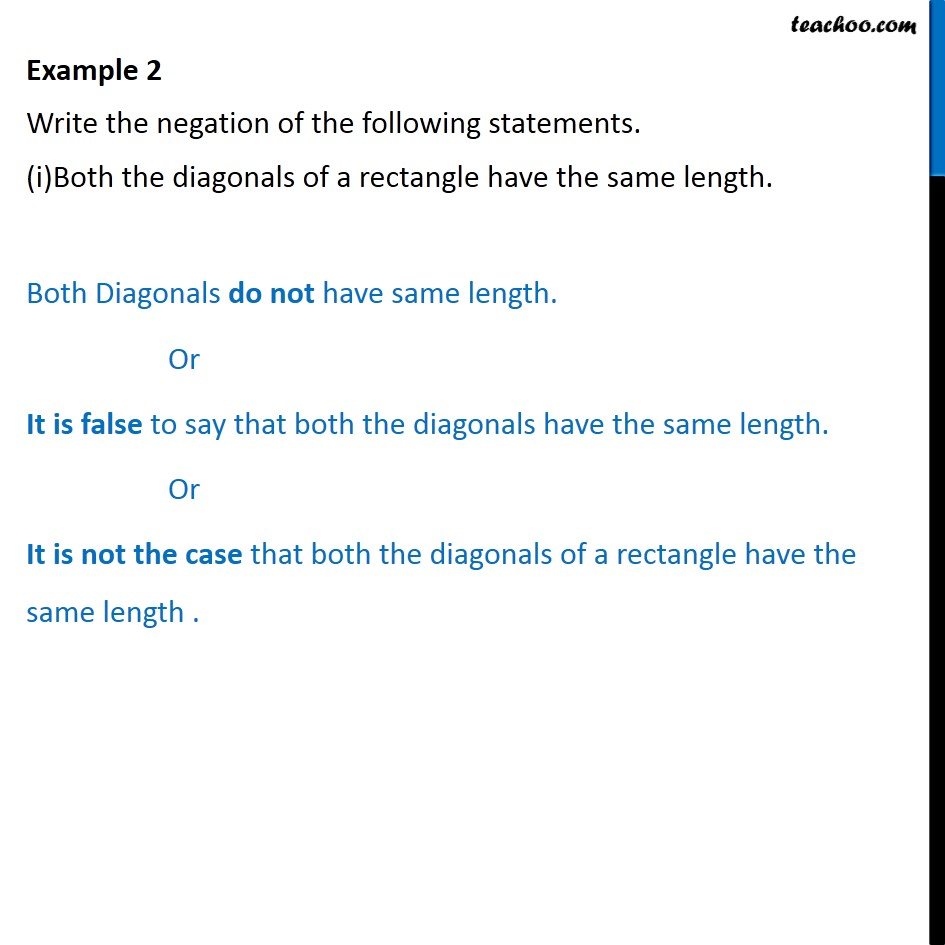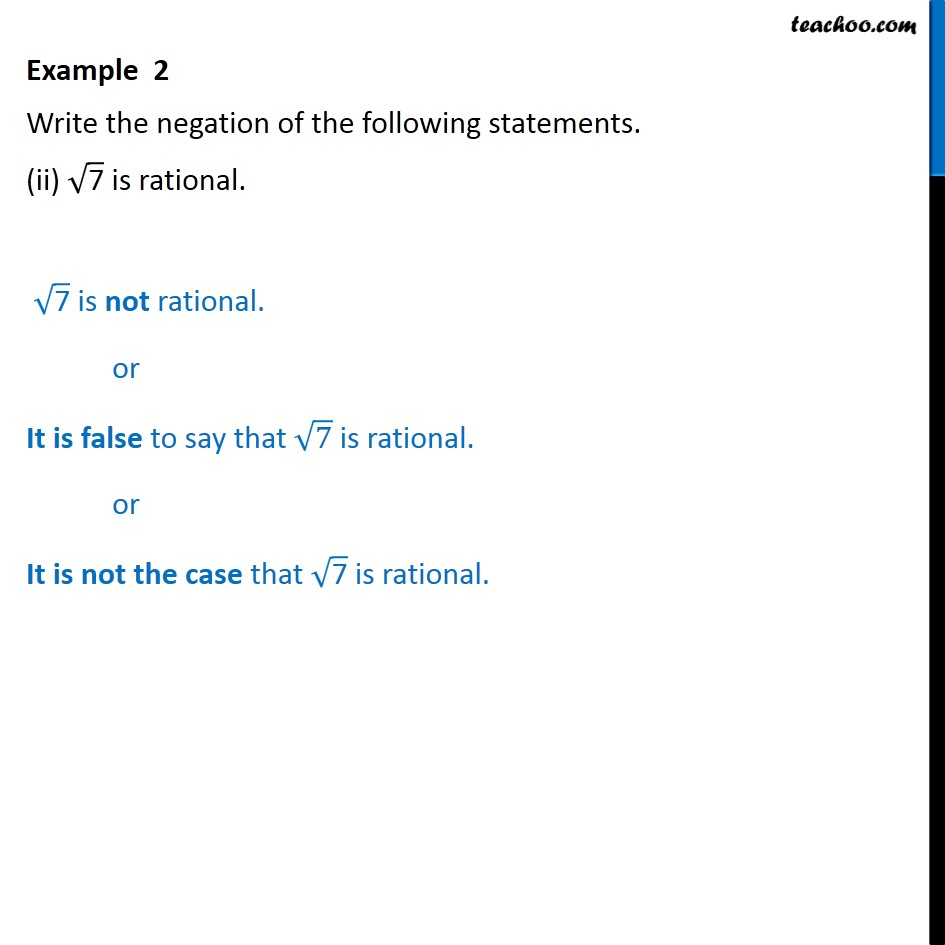Subscribe to our Youtube Channel - https://you.tube/teachoo

1. Chapter 14 Class 11 Mathematical Reasoning
2. Serial order wise
3. Examples

Transcript

Example 2 Write the negation of the following statements. (i)Both the diagonals of a rectangle have the same length. Both Diagonals do not have same length. Or It is false to say that both the diagonals have the same length. Or It is not the case that both the diagonals of a rectangle have the same length . Example 2 Write the negation of the following statements. (ii) 7 is rational. 7 is not rational. or It is false to say that 7 is rational. or It is not the case that 7 is rational.

Examples